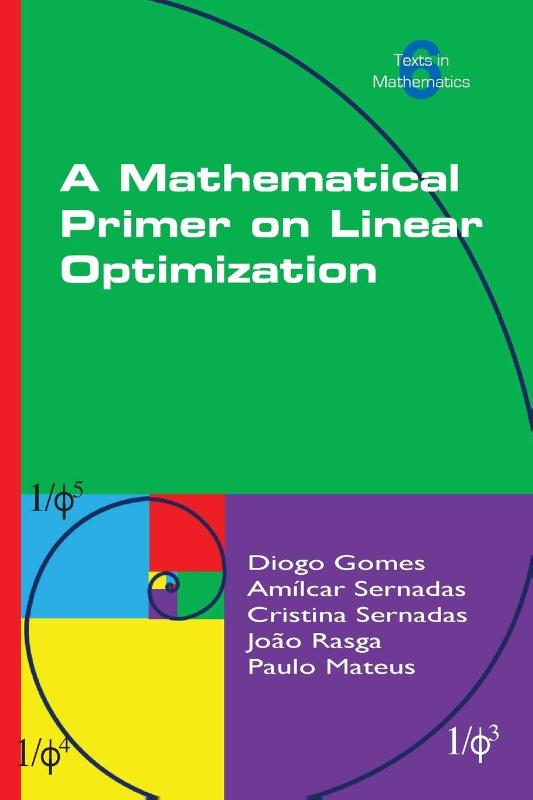# A Mathematical Primer on Linear Optimization

Taal: Engels

## € 20,95

Boeken gratis bezorgd vanaf € 15,- binnen NL en BE
Altijd de laagste prijs voor nieuwe Nederlandstalige boeken
Ruilen of retourneren binnen 14 dagen

The book provides a self-contained mathematical introduction to linear optimization for undergraduate students of Mathematics. This book is equally suitable for Science, Engineering, and Economics students who are interested in gaining a deeper understanding of the mathematical aspects of the subject. The linear optimization problem is analyzed from different perspectives: topological, algebraic, geometrical, logical, and algorithmic. Nevertheless, no previous knowledge of these subjects is required. The essential details are always provided in a special section at the end of each chapter. The technical material is illustrated with multiple examples, problems with fully-worked solutions, and a range of proposed exercises.

In Chapter 1, several formulations of the linear optimization problem are presented and related concerning admissible vectors and optimizers. Then, sufficient conditions for the existence of optimizers based on topological techniques are discussed in Chapter 2. The main objective of Chapter 3 is to provide a way for deciding whether or not an admissible vector is an optimizer, relying on Farkas' Lemma. In Chapter 4, linear algebra is used for computing optimizers via basic admissible vectors. A geometrical characterization of these vectors is the goal of Chapter 5. Duality is discussed in Chapter 6, giving yet a new technique for finding optimizers. An introduction to computational complexity is presented in Chapter 7 with the aim to analyze the efficiency of linear optimization algorithms. The complexity of a brute-force algorithm is shown not to be polynomial. Chapter 8 is targeted at the Simplex Algorithm. It includes the proof of its soundness and completeness and an explanation on its non-polynomial complexity. Finally, Chapter 9 concentrates on the integer optimization problem with an emphasis on totally unimodularity. An algorithm based on the Branch and Bound Technique is analyzed.
ISBN
9781848903159
Vorm
Paperback
Uitgever
College Publications
Taal
Engels
Pagina's
266 pp.
Genre
Geografie & Landbouw
Geen recensies beschikbaar.